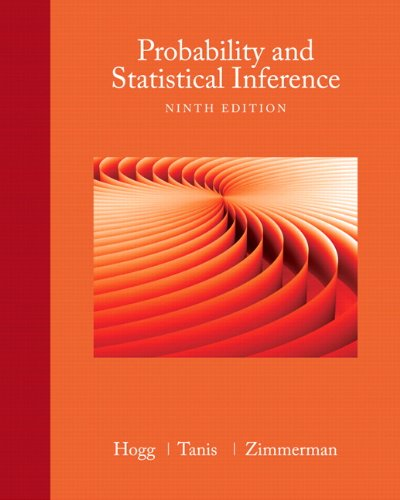#Reddit reviews Probability and Statistical Inference (9th Edition)

We found 6 Reddit comments about Probability and Statistical Inference (9th Edition). Here are the top ones, ranked by their Reddit score.

Probability & Statistics
Applied Mathematics
Mathematics
Science & Math
BooksWritten by three veteran statisticians, this applied introduction to probability and statistics emphasizes the existence of variation in almost every process, and how the study of probability and statistics helps us understand this variation.
Check price on Amazon

u/krtcl · 24 pointsr/learnmachinelearning

I've wasted too much time trying to find the so-called "right" statistics book. I'm still early in my journey, going through calculus using Prof. Leonards videos while working through a Linear Algebra book all in prep for tackling a stats book. Here's a list of books that I've had a look at so far.

&amp;#x200B;

• Probability and Statistical Inference by Hogg, Tanis and Zimmerman
• Mathematical Statistics with Applications by Wackerly

These seem to be of a similar level with regards to rigour, as they aren't that rigourous. That's not to say you can get by without the calculus prereq and even linear algebra

&amp;#x200B;

The other two I've been looking at which seem to be a lot more complex are

• Introduction to Mathematical Statistics by Hogg as well. I'd think it's the more rigorous version of the book mentioned above by the same author
• All of Statistics by Wasserman which seems to require a lot of prior knowledge in statistics, but I think tackles just the perfect topics for machine learning

And then there's Casella and Berger's Statistical inference, which I looked at once and decided not to look at again until I can manage at least one of the aforementioned books. I think I'm leaning most to the first book listed. Whichever one you decide to use, good luck with your journey.

&amp;#x200B;
u/mathnstats · 2 pointsr/statistics

Did any of your calc classes include multivariate/vector calculus? E.g. things dealing with double and triple integrals.

If not, take another calc class or two; calculus is very important for statistics. It shouldn't be too hard to pick up the rest of the necessary calc since you've already got a good calc background.

If so, start taking probability and statistics courses in your school's math department if you can. The mathematical way (read: the right way) of understanding probability and statistics is based on probability distributions (like the normal distribution), defined by their probability functions. As such, you can use calculus to obtain a myriad of information from them! For instance, among many other things, within the first one or 2 courses, you'd likely be able to answer at least the Spearman's coefficient question, the Bernoulli process question, and the MLE question.

If you don't have room in your schedule to do the stats course, you could get a textbook and try learning on your own. There are tons of excellent resources. Hogg, Tanis, and Zimmerman is pretty good for an introduction, though I'm sure there's better out there.

I can't remember, honestly. That's how bad it was. I'll dig out my notes tomorrow.

Prob Theory and Math Stats together was basically this book.

Ah, this is great! Thank you :)

I didn't manage to find the book I need for MATH 3215 though. Is there any way you could get Probability and Statistical Inference, Ninth Edition by Hogg, Tanis and Zimmerman?

u/Bomb3213 · 1 pointr/statistics

This imo is a good book for basic probability and mathematical statistics. Super easy read with a lot of examples. [You also mentioned pdf's for books and someone told you library gensis. I can promise this one is on there :)]

u/NegativeNail · 1 pointr/statistics

PDF WARN: Introduction to Math Stat by Hogg

Not to be confused with Probability and Math Stat by Tannis and Hogg which is a "first semester" course.

Good blend of theory and "talky-ness", good exercises that test your understanding, most should be do-able from just applying the basics.